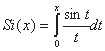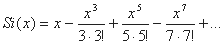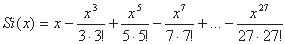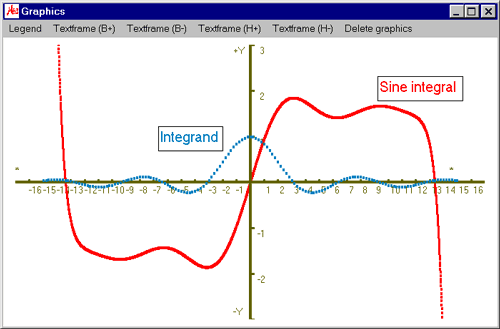# MasterAllRound: the PC calculator

## Plot module: example sine integral

The example below shows the application of factorials, constants and sub-equations:

The definite integralis the so-called sine integral withAs the equation Si(x) is quite long, we use sub-equations:
• t1= x - x^3/( 3*3! ) + x^5/( 5*5! ) - x^7/( 7*7!) + x^9/( 9*9! )
• t2= - x^11/( 11*11! ) + x^13/( 13*13! ) - x^15/( 15*15!) + x^17/( 17*17! )
• t3= - x^19/( 19*19! ) + x^21/( 21*21! ) - x^23/( 23*23!) + x^25/( 25*25! ) - x^27/( 27*27! )
The next steps are:
• We declare the variable and enter start value, end value and step width. We intend to plot the graph from -15 ≤ x ≤ +15. Note that the range of validity is dependent on the chosen number of terms. You will see that, in this case, the validity range is samller than the graph interval.
• Variable: x
• Start value: -15
• End value: 15
• Step width: 0.01
• We scale manually as below:
• X-axis, left: 17
• X-axis, right: 17
• Y-axis, top: 3
• Y-axis, bottom: 3
• And enter the function for Si(x):
• Sineintegral = t1 + t2 + t3 '@

We finalize the input with the return key and the graph of the function is then plotted: